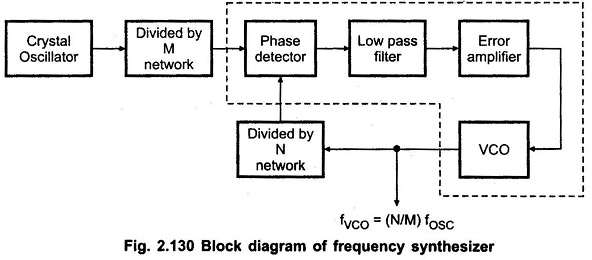## Block Diagram of Frequency Synthesizer using PLL:

The Block Diagram of Frequency Synthesizer using PLL that can produce a precise series of frequencies that are derived from a stable crystal controlled oscillator.The Fig. 2.130 shows the Frequency Synthesizer Block Diagram. It is similar to frequency multiplier circuit except that divided by M network is added at the input of phase lock loop.

The frequency of the crystal-controlled oscillator is divided by an integer factor M by divider network to produce a frequency fosc/M, where fosc is the frequency of the crystal controlled oscillator.

The VCO frequency fVCO is similarly divided by factor N by divider network to give frequency equal to fvco/N. When the PLL is locked in on the divided-down oscillator frequency, we will have fosc/M = fvco/N, so that fvco=(N/M)fosc.

By adjusting divider counts to desired values large number of frequencies can be produced, all derived from the crystal controlled oscillator.

Scroll to Top